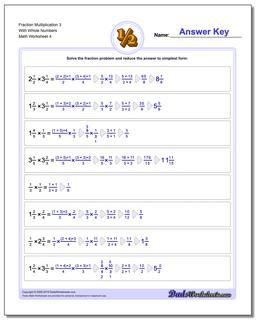# Math Worksheets: Fraction Multiplication: Fraction Multiplication: Fraction Multiplication 3 With Whole Numbers (Fourth Worksheet)## Fraction Multiplication 3 With Whole Numbers (Fourth Worksheet)

PropertyValue
DescriptionFraction Multiplication 3 With Whole Numbers: Basic fraction multiplication with whole parts, no cross-cancelling (Fourth Worksheet)
Resource TypeWorksheet